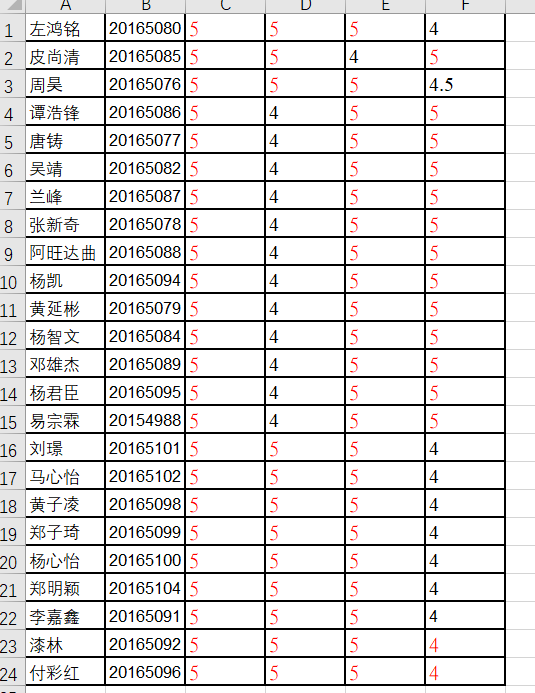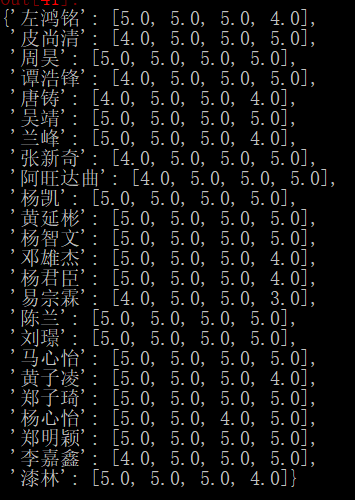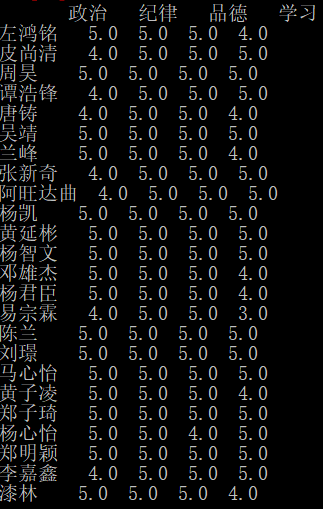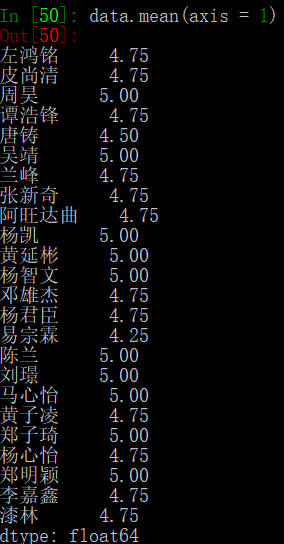• 2020-11-30 09:28:59

我正在计算我保存在文件夹中的大约50个excel文件的x、y和z列的平均值和标准值。每个excel文件的第一列有x值，第二列有y值，第三列有z值。我使用这个脚本，但它一直给我错误。所有文件都保存为“.xls”。如果你们能帮上忙的话，我可以帮上忙。以下是脚本和错误：import xlrd

import numpy

import os

path = "E:\\hello\\Patient"

dirList=os.listdir(path)

f = open('E:\\hello\\try.xls', 'w')

f.write('Patient_ID, Xavg, xstd, yavg, ystd, zavg, ystd')

f.write("\n")

##print dirList

##i = 0

Col_values=[]

for file in dirList:

fullpath = os.path.join(path,file)

## print fullpath

if os.path.isfile(fullpath) == 1:

wb = xlrd.open_workbook(fullpath)

sh = wb.sheet_by_index(0)

f.write(str(file))

f.write(", ")

for i in range(0,3):

for j in range(sh.nrows):

Col_values.append(sh.cell(j,i).value)

a = numpy.average(Col_values)

b = numpy.std(Col_values)

f.write(str(a))

f.write(", ")

f.write(str(b))

f.write(", ")

f.write("\n")

f.close()

更多相关内容
• ###################################################### python计算excel平均值和标准差 #####################################################‘‘‘:数据源格式编号时间仪器1仪器2仪器3仪器4仪器5仪器6仪器7...

##############################################

######## python计算excel平均值和标准差 #######

##############################################

‘‘‘:数据源格式

编号时间仪器1仪器2仪器3仪器4仪器5仪器6仪器7仪器8仪器9仪器10分组均值标准差

FKQXK-r-02-0120200702100101102103104144106107108201A组

FKQXK-r-02-0220200702100105102103104105106107108202A组

FKQXK-r-02-0320200702100101111151104105117107108203A组

‘‘‘

引入库

import xlwings as xw

import xlrd

import math

wb = xw.Book(‘伽玛数据处理.xls‘) #这样的话就不会频繁打开新的Excel

引用Excel工作表，单元格

引用工作表

sht = wb.sheets #sht = wb.sheets[第一个sheet名]====伽玛原始记录

引用单元格

rng = sht.range(‘a1‘) #rng = sht[‘a1‘] #rng = sht[0,0] 第一行的第一列即a1,相当于pandas的切片

定义函数

def mean(list): #定义平均值函数

sum=0

j=len(list)

for i in list:

sum = sum+ int(i)

return sum/j

def StdEv(list): #计算标准差函数，参数是列表和平均值

sum = 0.0

n = len(list) #计算列表长度

for i in list:

sum = sum + int(i)

list_aver=sum / n #先计算平均值

sum1 = 0.0

for j in range(n):

sum1 += (list[j] - list_aver) ** 2 #差的平方

res = math.sqrt(sum1/n) #求开方后就是标准差

return res

pass

上面定义函数

引用区域

rng = sht.range(‘c2:l2‘)

rng = sht[‘c‘]

n2=sht[‘n2‘]

list= sht.range(‘c2:l2‘).value

print(list)

print(mean(list))

sht.range(‘n2‘).value = mean(sht.range(‘c2:l2‘).value)

rng = sht.range(‘a2‘).expand(‘table‘)

nrows = rng.rows.count #获取总行数，不含标题

for i in range(2,nrows+2): #nrows+2是因为总行数不含标题，且最后一个不循环

sht.range(‘n{}‘.format(i)).value = mean(sht.range(‘c{}:l{}‘.format(i,i)).value) #计算均值列

sht.range(‘o{}‘.format(i)).value = StdEv(sht.range(‘c{}:l{}‘.format(i,i)).value) #计算标准差列

保存工作簿

wb.save(‘伽玛数据处理_RESULT.xls‘)

退出工作簿(可省略)

wb.close()

#######end###############

原文：https://www.cnblogs.com/quezesheng/p/13323761.html

展开全文• 使用python3读取处理excel表的数据内容如何对内容求平均值先说下概述：值就是集合平均数。(a1 a2 ……an)/n为a1，a2，……，an术平均值。简单算均数。有这么一组数字10、20、30、40、50那么它们的算术平均值是(10 20...

使用python3读取处理excel表的数据内容如何对内容求平均值

先说下概述：

值就是集合平均数。

(a1 a2 ……an)/n为a1，a2，……，an术平均值。

简单算均数。有这么一组数字10、20、30、40、50

那么它们的算术平均值是(10 20 30 40 50)/5=30

所以：

平均值=算术平均值

你还求什么？不用了呀！

如果在excel中求平均值可以用=average(e11:e15)

用python读取excel，怎样显示要查找的数据？

还是CSV格式的好处理一些，EXCEL的要装插件，以CSV为例，给一码

sqlserver

char

对c#

import

linecache

o=open('d:\\test\\14.csv')#文件路径

o.seek(0)

s1=str(input('输定位的信息:'))

a=0

...展开感觉还是CSV格式的好处理一些，EXCEL的插件，以CSV为例，给一段代码

sqlserver

char

对应c#

import

linecache

o=open('d:\\test\\14.csv')#文件路径

o.seek(0)

s1=str(input('输入要定位的信息:'))

a=0

count=0

for

i

in

r:

#按和值组合查找

if

i.find(s1)>=0:

print

r[a-1]#显示上一行

print

i#显示定位行

print

r[a 1]#显示下一行

a =1

print

'Total:%d'%count

例：

在以下数据中，查找含有T的行，并显示上一行(n行)

or

下一行(n行)

1，2，3，4

W,R,GFR,T

12,,F,34,G收起

python：提取txt文件中特定内容后的数据到excel

首先，你的TXT文件格式是否清晰，如果是类似表格的话，假如IPC在每行第三个位置，你可以按行读取，然后split剪切，得到IPC数据

最好把TXT贴上来比较好

python怎么从excel中读取数据？

VLOOKUP是一个查找函数，给定一个查找的目标，它从指定的查找区域中查回想要查找值。它的基本语法为：

VLOOKUP(查找目标，查找范围，返回值的列数，精确OR模糊查找)

下面以一个实例来介绍一下这四个参数的使用

例1：如下图所示，要求根据表二中的姓名，查找姓名所对应的年龄。

公式：B13 =VLOOKUP(A13,$B$2:$D$8,3,0)

参数说明：

1 查找目标：就是你指定的查找的内容或单元格引用。本例中表二A列的姓名就是查找目标。我们要根据表二的“姓名”在表一中A列进行查找。

公式：B13 =VLOOKUP(A13,$B$2:$D$8,3,0)

2 查找范围(VLOOKUP(A13,$B$2:$D$8,3,0) )：指定了查找目标，如果没有说从哪里查找，EXCEL肯定会很为难。所以下一步我们就要指定从哪个范围中进行查找。VLOOKUP的这第二个参数可以从一个单元格区域中查找，也可以从一个常量数组或内存数组中查找。本例中要从表一中进行查找，那么范围我们要怎么指定呢？这里也是极易出错的地方。大家一定要注意，给定的第二个参数查找范围要符合以下条件才不会出错：

A 查找目标一定要在该区域的第一列。本例中查找表二的姓名，那么姓名所对应的表一的姓名列，那么表一的姓名列(列)一定要是查找区域的第一列。象本例中，给定的区域要从第二列开始，即$B$2:$D$8，而不能是$A$2:$D$8。因为查找的“姓名”不在$A$2:$D$8区域的第一列。

B 该区域中一定要包含要返回值所在的列，本例中要返回的值是年龄。年龄列(表一的D列)一定要包括在这个范围内，即：$B$2:$D$8，如果写成$B$2:$C$8就是错的。

3 返回值的列数(B13 =VLOOKUP(A13,$B$2:$D$8,3,0))。这是VLOOKUP第3个参数。它是一个整数值。它怎么得来的呢。它是“返回值”在第二个参数给定的区域中的列数。本例中我们要返回的是“年龄”，它是第二个参数查找范围$B$2:$D$8的第3列。这里一定要注意，列数不是在工作表中的列数(不是第4列)，而是在查找范围区域的第几列。如果本例中要是查找姓名所对应的性别，第3个参数的值应该设置为多少呢。答案是2。因为性别在$B$2:$D$8的第2列中。

4 精确OR模糊查找(VLOOKUP(A13,$B$2:$D$8,3,0) )，最后一个参数是决定函数精确和模糊查找的关键。精确即完全一样，模糊即包含的意思。第4个参数如果指定值是0或FALSE就表示精确查找，而值为1 或TRUE时则表示模糊。这里兰色提醒大家切记切记，在使用VLOOKUP时千万不要把这个参数给漏掉了，如果缺少这个参数默为值为模糊查找，我们就无法精确查找到结果了。

1、接下来，我们的任务是通过利用VLOOKUP函数来实现查找同学C的成绩。为此在单元格中输入“=VLOOKUP”，此时就会发现VLOOKUP包括三个参数和一个可选参数。

其中“lookup_value”是指要查找的值。

参数“table_array”是指搜索的区域，在此在除标题之后的整个数据区域。

第三个参数“col_index_num”是指整个函数返回单元格所在的列号。

2、最后以右括号结尾，并按回车键，就出现想要的结果啦。

python 如何读取excel文件 将每一行存为数组

from xlrd import open_workbook

wb=open_workbook(r'd:/222.xlsx')

tb=wb.sheets()

data=[]

for r in range(tb.nrows):

val=[]

for c in range(tb.ncols):

val.append(tb.cell_value(r,c))

data.append(tuple(val))

print(data)

展开全文• 问题遇到的现象和发生背景 计算numbers.xlsx中的每一行的平均值，并添加到numbers.xlsx 表中最右侧的列中。计算每一列的数据之和，添加在每一列的最下方。 最终计算行和列的总和，填写在右下角的单元格中。 问题相关...
• 使用ipython进行代码编写，清洗excel的数据并且转化到DataFrame上进行平均值的输出，最后输出为新的Excel的表格

# Python读取Excel文件取平均数

## 1.文件读取

下面我们将对这个excel文件进行读取import xlrd                           #xlrd是excel文件读取库 只读写
data = xlrd.open_workbook('a.xlsx')   #打开excel文件
table = data.sheets()              #打开第一张表格
nrows = table.nrows                   #获取表格的行数


接下来利用For循环对每行进行处理，处理的目的是把想要的数据存储在列表当中

for i in range(nrows):
name_.append(table.row_values(i))
data_.append(table.row_values(i)[2:5])


## 2.整合为字典

这一步是获取数据后第一个要处理的关键
初始化字典的方法有许多
但是最常用的方法还是使用
zip
可以把两个对应位置的列表进行整合为字典

dict_data = dict(zip(name_,data_))


输出结果再根据字典输出为DataFrame

data  = pd.DataFrame(dict_data,index=['政治','纪律','品德','学习'])


再进行转置输出

data.T## 2.平均数的读取

 data.mean(axis = 1)


mean（）里面axis表示的是坐标轴，axis = 0表示对纵向求平均值，axis = 1表示对横向求平均值展开全文文件操作
• # 读取excel表格 import xlrd from xlutils.copy import copy ## 第一步，读取 # excel表格在哪里 filename = "./students.xls" workbook = xlrd.open_workbook(filename) sheetnames = workbook.sheet_names() ...
• 首先把你的数据放到panda数据框中——我...(来自csv或excel)。启动框架import pandas as pdimport datetimedf1 = pd.DataFrame({'Start_date' : ['2018-01-01','2018-01-02','2018-01-03','2018-02-01','2018-03-10...
• Python实战：求“B列”、“C列“、“D列”平均值，此过程略有麻烦，请仔细观看下面步骤：'。1、核心代码2、运行结果3、过程分析此过程分为三个步骤，得到z1与z2两个变量，再将结果整合得到结果。3.1、z1变量的分解：...
• 我是Python新手，我在这个问题上遇到了很多麻烦，这是我工作中必须做的事情。关于excel文件的一些背景：有3列，大约100行。第一列(col1)包含A或B。第二列(col2)包含1到10之间的任意数字。第三列(col3)包含任意十进制...
• 绩点计算规则：（加权平均分-50）/10 故代码实现如下： 本质：求加权平均数 import numpy as np import pandas as pd score = pd.read_excel(r"C:\Users\Lenovo\Desktop\理想成绩.xlsx") print("加权平均分为：") ...numpy
• 需求：需要取某列数据在相同时间段内的平均值，比如：每5min取一次。 思路：读入的数据格式为DataFrame，先通过 df['某列'][ i:j] 的形式取数，用循环可以依次取数成Serise格式，循环里面用append函数将每次循环值...list
• 那是 cas diff dist ind 0 1 0.54 10 la 1 2 3.20 5 p 2 3 8.60 7 la 3 4 7.20 8 la 4 5 2.10 7 p 5 6 1.00 2 g 6 7 3.50 5 g 7 8 4.50 3 la 我需要计算权重在’dist’列中的所有列的加权平均值,并将值分组为’ind’...
• 我们要计算每个工作簿的工作表中值的总和与平均值，写入原文件(这里先复制出一份和原来一样的文件后写入新文件) 知识点如下：pandas的函数、读取多个工作簿下的多个工作表、同时向一个Excel文件内写入多个sheet，一...
• from __future__ import divisionimport csvfrom collections import defaultdictdef default_factory():return [0, None, None, 0]reader = csv.DictReader(open(r'snow_data.csv'))dates = defaultdict(default_f....
• Python实现按某一列关键字分组，并计算各列的平均值，并用该值填充该分类该列的nan值。 DataFrame数据格式 fillna方式实现 groupby方式实现 DataFrame数据格式 以下是数据存储形式： fillna方式实现 1、按照...
• “臭氧8小时”是一个滑动平均值，是将0-23小时中从0-7开始每8小时计算一个均值作为7时的值，1-8计算的均值作为8时的值，以此类推滑动计算。 总结 做到了使用groupby按天分组，计算每天数据的滑动平均。如下，主要...
• 2017-01-20 回答读excel要用到xlrd模块，官网安装（http://pypi.python.org/pypi/xlrd）。然后就可以跟着里面的例子稍微试一下就知道怎么用了。大概的流程是这样的：1、导入模块import xlrd2、打开excel文件读取数据...
• ## 在Python上求平均值

千次阅读 2021-01-11 19:47:55
字段[Num]中在(3987)和(5026)范围内的数字的平均值是多少？在下面的字段是excel中的字段。在**amount code quan val number Random**2.11 I[N8U7]:75 184 Blue 2254 Potato3.13 Z[V0L8]:64 131 Blue 6349 Carro.....
• 本文介绍使用ExcelPython计算上述统计，而Hive和Spark将放在下一篇中。1、数据介绍这里咱们使用的是iris分类数据集，数据下载地址为：http://archive.ics.uci.edu/ml/datasets/Iris下载后转换...
•   又到了一年一度的算综测时间，其中一大难点就是计算全班同学相互打分的平均值；而若借助Python，这一问题便迎刃而解。   目前有一个文件夹，其中存放了大量Excel文件；文件名称是每一位同学的名字，即文件名称...xlsx ms office openpyxl
• 输出:把RGB显示到标签上，第一个数字并保留两位小数 import os import xml.dom.minidom import cv2 import numpy as np from tqdm import tqdm import random from ipdb import set_trace from IPython import ...开发语言 后端
• python求加权平均值的实例(附纯python写法)首先是数据源：#需要求加权平均值的数据列表elements = []#对应的权值列表weights = []使用numpy直接求：import numpy as npnp.average(elements, weights=weights)附纯...
• 如何使用python或者宏对excel中数据进行统计重复次数这会写这样的代码！要看你具体的Excel文档，不好妄自揣测。(如果不方便可以根据实际情况模拟数据)python 如何读取 excel 指定单元格内容1、首先在filepathName = ......